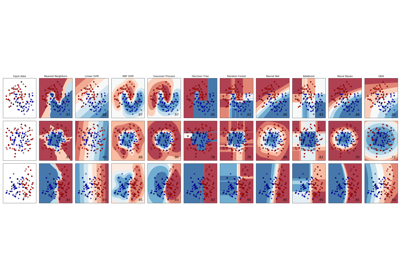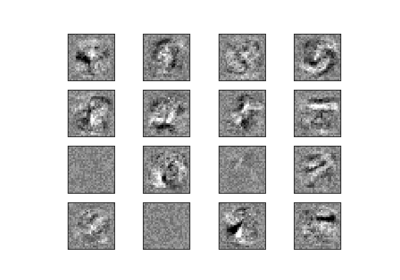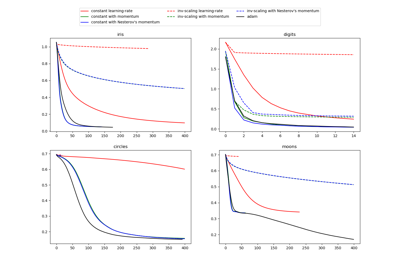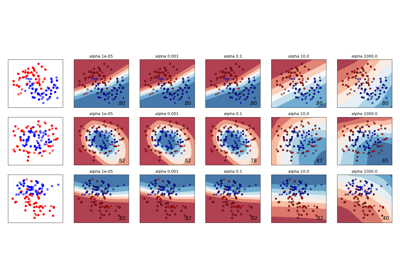# sklearn.neural_network.MLPClassifier¶

class sklearn.neural_network.MLPClassifier(hidden_layer_sizes=(100, ), activation='relu', solver='adam', alpha=0.0001, batch_size='auto', learning_rate='constant', learning_rate_init=0.001, power_t=0.5, max_iter=200, shuffle=True, random_state=None, tol=0.0001, verbose=False, warm_start=False, momentum=0.9, nesterovs_momentum=True, early_stopping=False, validation_fraction=0.1, beta_1=0.9, beta_2=0.999, epsilon=1e-08, n_iter_no_change=10)[source]

Multi-layer Perceptron classifier.

This model optimizes the log-loss function using LBFGS or stochastic gradient descent.

New in version 0.18.

Parameters: hidden_layer_sizes : tuple, length = n_layers - 2, default (100,) The ith element represents the number of neurons in the ith hidden layer. activation : {‘identity’, ‘logistic’, ‘tanh’, ‘relu’}, default ‘relu’ Activation function for the hidden layer. ‘identity’, no-op activation, useful to implement linear bottleneck, returns f(x) = x ‘logistic’, the logistic sigmoid function, returns f(x) = 1 / (1 + exp(-x)). ‘tanh’, the hyperbolic tan function, returns f(x) = tanh(x). ‘relu’, the rectified linear unit function, returns f(x) = max(0, x) solver : {‘lbfgs’, ‘sgd’, ‘adam’}, default ‘adam’ The solver for weight optimization. ‘lbfgs’ is an optimizer in the family of quasi-Newton methods. ‘sgd’ refers to stochastic gradient descent. ‘adam’ refers to a stochastic gradient-based optimizer proposed by Kingma, Diederik, and Jimmy Ba Note: The default solver ‘adam’ works pretty well on relatively large datasets (with thousands of training samples or more) in terms of both training time and validation score. For small datasets, however, ‘lbfgs’ can converge faster and perform better. alpha : float, optional, default 0.0001 L2 penalty (regularization term) parameter. batch_size : int, optional, default ‘auto’ Size of minibatches for stochastic optimizers. If the solver is ‘lbfgs’, the classifier will not use minibatch. When set to “auto”, batch_size=min(200, n_samples) learning_rate : {‘constant’, ‘invscaling’, ‘adaptive’}, default ‘constant’ Learning rate schedule for weight updates. ‘constant’ is a constant learning rate given by ‘learning_rate_init’. ‘invscaling’ gradually decreases the learning rate at each time step ‘t’ using an inverse scaling exponent of ‘power_t’. effective_learning_rate = learning_rate_init / pow(t, power_t) ‘adaptive’ keeps the learning rate constant to ‘learning_rate_init’ as long as training loss keeps decreasing. Each time two consecutive epochs fail to decrease training loss by at least tol, or fail to increase validation score by at least tol if ‘early_stopping’ is on, the current learning rate is divided by 5. Only used when solver='sgd'. learning_rate_init : double, optional, default 0.001 The initial learning rate used. It controls the step-size in updating the weights. Only used when solver=’sgd’ or ‘adam’. power_t : double, optional, default 0.5 The exponent for inverse scaling learning rate. It is used in updating effective learning rate when the learning_rate is set to ‘invscaling’. Only used when solver=’sgd’. max_iter : int, optional, default 200 Maximum number of iterations. The solver iterates until convergence (determined by ‘tol’) or this number of iterations. For stochastic solvers (‘sgd’, ‘adam’), note that this determines the number of epochs (how many times each data point will be used), not the number of gradient steps. shuffle : bool, optional, default True Whether to shuffle samples in each iteration. Only used when solver=’sgd’ or ‘adam’. random_state : int, RandomState instance or None, optional, default None If int, random_state is the seed used by the random number generator; If RandomState instance, random_state is the random number generator; If None, the random number generator is the RandomState instance used by np.random. tol : float, optional, default 1e-4 Tolerance for the optimization. When the loss or score is not improving by at least tol for n_iter_no_change consecutive iterations, unless learning_rate is set to ‘adaptive’, convergence is considered to be reached and training stops. verbose : bool, optional, default False Whether to print progress messages to stdout. warm_start : bool, optional, default False When set to True, reuse the solution of the previous call to fit as initialization, otherwise, just erase the previous solution. See the Glossary. momentum : float, default 0.9 Momentum for gradient descent update. Should be between 0 and 1. Only used when solver=’sgd’. nesterovs_momentum : boolean, default True Whether to use Nesterov’s momentum. Only used when solver=’sgd’ and momentum > 0. early_stopping : bool, default False Whether to use early stopping to terminate training when validation score is not improving. If set to true, it will automatically set aside 10% of training data as validation and terminate training when validation score is not improving by at least tol for n_iter_no_change consecutive epochs. Only effective when solver=’sgd’ or ‘adam’ validation_fraction : float, optional, default 0.1 The proportion of training data to set aside as validation set for early stopping. Must be between 0 and 1. Only used if early_stopping is True beta_1 : float, optional, default 0.9 Exponential decay rate for estimates of first moment vector in adam, should be in [0, 1). Only used when solver=’adam’ beta_2 : float, optional, default 0.999 Exponential decay rate for estimates of second moment vector in adam, should be in [0, 1). Only used when solver=’adam’ epsilon : float, optional, default 1e-8 Value for numerical stability in adam. Only used when solver=’adam’ n_iter_no_change : int, optional, default 10 Maximum number of epochs to not meet tol improvement. Only effective when solver=’sgd’ or ‘adam’ New in version 0.20. classes_ : array or list of array of shape (n_classes,) Class labels for each output. loss_ : float The current loss computed with the loss function. coefs_ : list, length n_layers - 1 The ith element in the list represents the weight matrix corresponding to layer i. intercepts_ : list, length n_layers - 1 The ith element in the list represents the bias vector corresponding to layer i + 1. n_iter_ : int, The number of iterations the solver has ran. n_layers_ : int Number of layers. n_outputs_ : int Number of outputs. out_activation_ : string Name of the output activation function.

Notes

MLPClassifier trains iteratively since at each time step the partial derivatives of the loss function with respect to the model parameters are computed to update the parameters.

It can also have a regularization term added to the loss function that shrinks model parameters to prevent overfitting.

This implementation works with data represented as dense numpy arrays or sparse scipy arrays of floating point values.

References

Hinton, Geoffrey E.
“Connectionist learning procedures.” Artificial intelligence 40.1 (1989): 185-234.
Glorot, Xavier, and Yoshua Bengio. “Understanding the difficulty of
training deep feedforward neural networks.” International Conference on Artificial Intelligence and Statistics. 2010.
He, Kaiming, et al. “Delving deep into rectifiers: Surpassing human-level
performance on imagenet classification.” arXiv preprint arXiv:1502.01852 (2015).
Kingma, Diederik, and Jimmy Ba. “Adam: A method for stochastic
optimization.” arXiv preprint arXiv:1412.6980 (2014).

Methods

 fit(X, y) Fit the model to data matrix X and target(s) y. get_params([deep]) Get parameters for this estimator. predict(X) Predict using the multi-layer perceptron classifier predict_log_proba(X) Return the log of probability estimates. predict_proba(X) Probability estimates. score(X, y[, sample_weight]) Returns the mean accuracy on the given test data and labels. set_params(**params) Set the parameters of this estimator.
__init__(hidden_layer_sizes=(100, ), activation='relu', solver='adam', alpha=0.0001, batch_size='auto', learning_rate='constant', learning_rate_init=0.001, power_t=0.5, max_iter=200, shuffle=True, random_state=None, tol=0.0001, verbose=False, warm_start=False, momentum=0.9, nesterovs_momentum=True, early_stopping=False, validation_fraction=0.1, beta_1=0.9, beta_2=0.999, epsilon=1e-08, n_iter_no_change=10)[source]
fit(X, y)[source]

Fit the model to data matrix X and target(s) y.

Parameters: X : array-like or sparse matrix, shape (n_samples, n_features) The input data. y : array-like, shape (n_samples,) or (n_samples, n_outputs) The target values (class labels in classification, real numbers in regression). self : returns a trained MLP model.
get_params(deep=True)[source]

Get parameters for this estimator.

Parameters: deep : boolean, optional If True, will return the parameters for this estimator and contained subobjects that are estimators. params : mapping of string to any Parameter names mapped to their values.
partial_fit

Update the model with a single iteration over the given data.

Parameters: X : {array-like, sparse matrix}, shape (n_samples, n_features) The input data. y : array-like, shape (n_samples,) The target values. classes : array, shape (n_classes), default None Classes across all calls to partial_fit. Can be obtained via np.unique(y_all), where y_all is the target vector of the entire dataset. This argument is required for the first call to partial_fit and can be omitted in the subsequent calls. Note that y doesn’t need to contain all labels in classes. self : returns a trained MLP model.
predict(X)[source]

Predict using the multi-layer perceptron classifier

Parameters: X : {array-like, sparse matrix}, shape (n_samples, n_features) The input data. y : array-like, shape (n_samples,) or (n_samples, n_classes) The predicted classes.
predict_log_proba(X)[source]

Return the log of probability estimates.

Parameters: X : array-like, shape (n_samples, n_features) The input data. log_y_prob : array-like, shape (n_samples, n_classes) The predicted log-probability of the sample for each class in the model, where classes are ordered as they are in self.classes_. Equivalent to log(predict_proba(X))
predict_proba(X)[source]

Probability estimates.

Parameters: X : {array-like, sparse matrix}, shape (n_samples, n_features) The input data. y_prob : array-like, shape (n_samples, n_classes) The predicted probability of the sample for each class in the model, where classes are ordered as they are in self.classes_.
score(X, y, sample_weight=None)[source]

Returns the mean accuracy on the given test data and labels.

In multi-label classification, this is the subset accuracy which is a harsh metric since you require for each sample that each label set be correctly predicted.

Parameters: X : array-like, shape = (n_samples, n_features) Test samples. y : array-like, shape = (n_samples) or (n_samples, n_outputs) True labels for X. sample_weight : array-like, shape = [n_samples], optional Sample weights. score : float Mean accuracy of self.predict(X) wrt. y.
set_params(**params)[source]

Set the parameters of this estimator.

The method works on simple estimators as well as on nested objects (such as pipelines). The latter have parameters of the form <component>__<parameter> so that it’s possible to update each component of a nested object.

Returns: self

## Examples using sklearn.neural_network.MLPClassifier¶Classifier comparisonVisualization of MLP weights on MNISTCompare Stochastic learning strategies for MLPClassifierVarying regularization in Multi-layer Perceptron